# NCERT Solutions for Class 10 Maths Exercise 4.2## myCBSEguide App

Complete Guide for CBSE Students

NCERT Solutions, NCERT Exemplars, Revison Notes, Free Videos, CBSE Papers, MCQ Tests & more.

NCERT solutions for Maths Quadratic Equations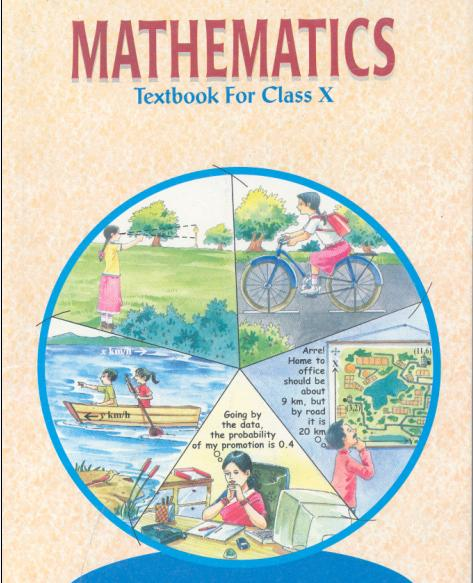## NCERT Solutions for Class 10 Maths Quadratic Equations

###### 1. Find the roots of the following Quadratic Equations by factorization.

(i)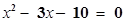(ii)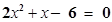(iii)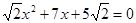(iv)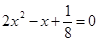(v)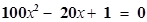Ans. (i)x2 − 3x – 10 = 0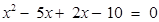x (x − 5) + 2 (x − 5) = 0

⇒(x − 5) (x + 2) = 0

x = 5, −2

(ii)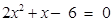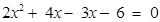⇒2x (x + 2) – 3 (x + 2) = 0

⇒(2x − 3) (x + 2) = 0

x =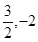(iii)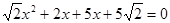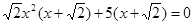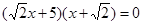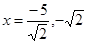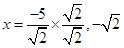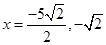(iv)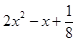= 0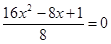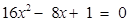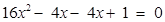⇒4x (4x − 1) – 1 (4x − 1) = 0

⇒(4x − 1) (4x − 1) = 0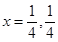(v)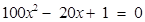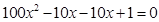⇒10x (10x − 1) – 1 (10x − 1) = 0

⇒(10x − 1) (10x − 1) = 0

x =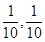NCERT Solutions for Class 10 Maths Exercise 4.2

###### 2. Solve the following problems given:

(i)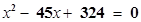(ii)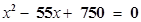Ans. (i)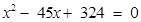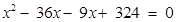x (x − 36) – 9 (x − 36) = 0

⇒(x − 9) (x − 36) = 0

x = 9, 36

(ii)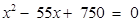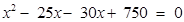x (x − 25) – 30 (x − 25) = 0

⇒(x − 30) (x − 25) = 0

x = 30, 25

NCERT Solutions for Class 10 Maths Exercise 4.2

###### 3.Find two numbers whose sum is 27 and product is 182.

Ans. Let first number be x and let second number be (27 − x)

According to given condition, the product of two numbers is 182.

Therefore,

x (27 − x) = 182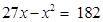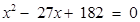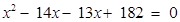x (x − 14) – 13 (x − 14) = 0

⇒(x − 14) (x − 13) = 0

x = 14, 13

Therefore, the first number is equal to 14 or 13

And, second number is = 27 – x = 27 – 14 = 13 or Second number = 27 – 13 = 14

Therefore, two numbers are 13 and 14.

NCERT Solutions for Class 10 Maths Exercise 4.2

###### 4. Find two consecutive positive integers, sum of whose squares is 365.

Ans. Let first number be x and let second number be (x + 1)

According to given condition,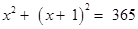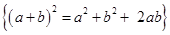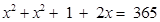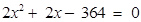Dividing equation by 2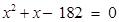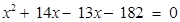x (x + 14) – 13 (x + 14) = 0

⇒ (x + 14) (x − 13) = 0

x = 13, −14

Therefore, first number = 13 {We discard -14 because it is negative number)

Second number = x + 1 = 13 + 1 = 14

Therefore, two consecutive positive integers are 13 and 14 whose sum of squares is equal to 365.

NCERT Solutions for Class 10 Maths Exercise 4.2

###### 5.The altitude of right triangle is 7 cm less than its base. If, hypotenuse is 13 cm. Find the other two sides.

Ans. Let base of triangle be x cm and let altitude of triangle be (x − 7) cm

It is given that hypotenuse of triangle is 13 cm

According to Pythagoras Theorem,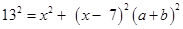=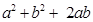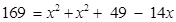⇒169 =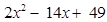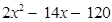= 0

Dividing equation by 2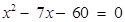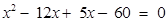x (x − 12) + 5 (x − 12) = 0

⇒(x − 12) (x + 5)

x = −5, 12

We discard x = −5 because length of side of triangle cannot be negative.

Therefore, base of triangle = 12 cm

Altitude of triangle = (x − 7) = 12 – 7 = 5 cm

NCERT Solutions for Class 10 Maths Exercise 4.2

###### 6. A cottage industry produces a certain number of pottery articles in a day. It was observed on a particular day that cost of production of each article (in rupees) was 3 more than twice the number of articles produced on that day. If, the total cost of production on that day was Rs. 90, find the number of articles produced and the cost of each article.

Ans. Let cost of production of each article be Rs x

We are given total cost of production on that particular day = Rs 90

Therefore, total number of articles produced that day = 90/x

According to the given conditions,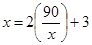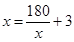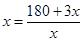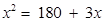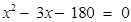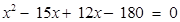x (x − 15) + 12 (x − 15) = 0

⇒(x − 15) (x + 12) = 0⇒x = 15, −12

Cost cannot be in negative, therefore, we discard x = −12

Therefore, x = Rs 15 which is the cost of production of each article.

Number of articles produced on that particular day =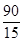= 6

## NCERT Solutions for Class 10 Maths Exercise 4.2

NCERT Solutions Class 10 Maths PDF (Download) Free from myCBSEguide app and myCBSEguide website. Ncert solution class 10 Maths includes text book solutions from Mathematics Book. NCERT Solutions for CBSE Class 10 Maths have total 15 chapters. 10 Maths NCERT Solutions in PDF for free Download on our website. Ncert Maths class 10 solutions PDF and Maths ncert class 10 PDF solutions with latest modifications and as per the latest CBSE syllabus are only available in myCBSEguide.

## CBSE app for Class 10

To download NCERT Solutions for Class 10 Maths, Computer Science, Home Science,Hindi ,English, Social Science do check myCBSEguide app or website. myCBSEguide provides sample papers with solution, test papers for chapter-wise practice, NCERT solutions, NCERT Exemplar solutions, quick revision notes for ready reference, CBSE guess papers and CBSE important question papers. Sample Paper all are made available through the best app for CBSE students and myCBSEguide website.## Test Generator

Create Papers with your Name & Logo

### 6 thoughts on “NCERT Solutions for Class 10 Maths Exercise 4.2”

1. Thanks

2. was so much helpfull to me

3. Thanku so much mycbseguide is very helpful

4. Thanku so much

5. very good